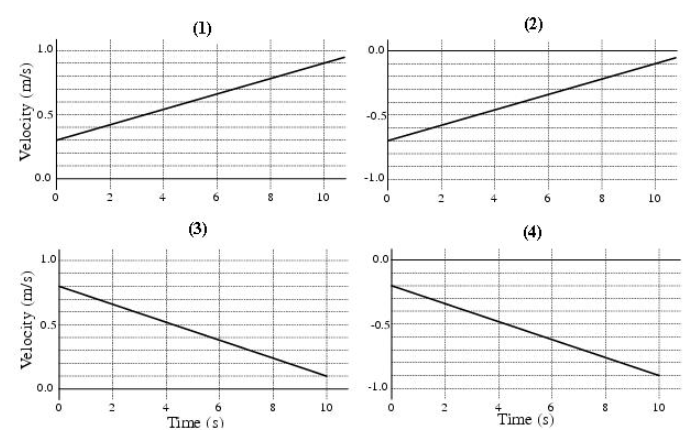# Problem: Which graphs represent an object that is slowing down? A. 2 and 3 B. 2 and 4 C. 1 and 4 D. 1 and 2 E. 1 and 3 F. 3 and 4

###### FREE Expert Solution

An object slows down when the magnitude of the velocity is decreasing.

84% (397 ratings)###### Problem Details

Which graphs represent an object that is slowing down?A. 2 and 3

B. 2 and 4

C. 1 and 4

D. 1 and 2

E. 1 and 3

F. 3 and 4